# HSSlive: Plus One & Plus Two Notes & Solutions for Kerala State Board

## BSEB Class 9 Maths Chapter 10 Circles Ex 10.5 Textbook Solutions PDF: Download Bihar Board STD 9th Maths Chapter 10 Circles Ex 10.5 Book AnswersBSEB Class 9 Maths Chapter 10 Circles Ex 10.5 Textbook Solutions PDF: Download Bihar Board STD 9th Maths Chapter 10 Circles Ex 10.5 Book Answers

BSEB Class 9th Maths Chapter 10 Circles Ex 10.5 Textbooks Solutions and answers for students are now available in pdf format. Bihar Board Class 9th Maths Chapter 10 Circles Ex 10.5 Book answers and solutions are one of the most important study materials for any student. The Bihar Board Class 9th Maths Chapter 10 Circles Ex 10.5 books are published by the Bihar Board Publishers. These Bihar Board Class 9th Maths Chapter 10 Circles Ex 10.5 textbooks are prepared by a group of expert faculty members. Students can download these BSEB STD 9th Maths Chapter 10 Circles Ex 10.5 book solutions pdf online from this page.

## Bihar Board Class 9th Maths Chapter 10 Circles Ex 10.5 Books Solutions

 Board BSEB Materials Textbook Solutions/Guide Format DOC/PDF Class 9th Subject Maths Chapter 10 Circles Ex 10.5 Chapters All Provider Hsslive

## How to download Bihar Board Class 9th Maths Chapter 10 Circles Ex 10.5 Textbook Solutions Answers PDF Online?

2. Click on the Bihar Board Class 9th Maths Chapter 10 Circles Ex 10.5 Answers.
3. Look for your Bihar Board STD 9th Maths Chapter 10 Circles Ex 10.5 Textbooks PDF.
4. Now download or read the Bihar Board Class 9th Maths Chapter 10 Circles Ex 10.5 Textbook Solutions for PDF Free.

Find below the list of all BSEB Class 9th Maths Chapter 10 Circles Ex 10.5 Textbook Solutions for PDF’s for you to download and prepare for the upcoming exams:

## BSEB Bihar Board Class 9th Maths Solutions Chapter 10 Circles Ex 10.5

Question 1.
In figure, A, B and C are three points on a circle with centre O such that ∠BOC = 30° and ∠AOB = 60°. If D is a point on the circle other than the arc ABC, find ∠ADC.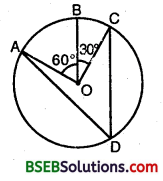Solution:
Since arc ABC makes ∠AOC = ∠AOB + ∠AOC = 60° + 30° = 90° at the centre of the circle and ∠ADC at a point on the remaining part of the circle.
∴ ∠ADC = 12(∠AOC) = 12 x 90° = 45°

Question 2.
A chord of a circle is equal to the radius of the circle. Find the angle subtended by the chord at a point on the minor arc and also at a point on the major arc.
Solution:
Let PQ be chord. Join OP and OQ.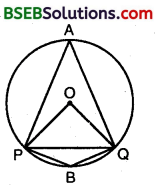It is given that PQ = OP = OQ (∵ Chord = radius)
∴ Δ OPQ is equilateral. A
⇒ ∠POQ = 60°
Since arc PBQ makes reflex ∠POQ = 360° – 60° = 300° at the centre of the circle and ∠PBQ at a point in the minor arc of the circle.Hence, angle subtended by the chord on the minor arc = 150° and on the major chord = 30°.

Question 3.
In figure, ∠PQR = 100°, where P, Q and R are points on a circle with centre O. Find ∠OPR.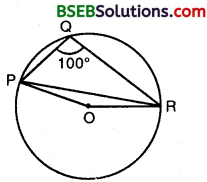Solution:
Since the angle subtended by an arc of a circle at its centre is twice the angle subtended by the same arc at a point on the circumference.
∴ Reflex ∠POR = 2∠PQR
⇒ Reflex ∠POR = 2 x 100° = 200°
⇒ ∠POR = 360° – 200° = 160°
In ∆ OPR, OP = OR [Radii of the same circle}
∠OPR = ∠ORP [Angles opp. to equal sides are equal]
and ∠POR = 160° [Proved above]
∴ ∠OPR = ∠ORP = 12(180° – 160°)
= 12 x 20° = 10°
Hence, ∠OPR = 10°.

Question 4.
In figure, ∠ABC = 69°, ∠ACB = 31°, find ∠BDC.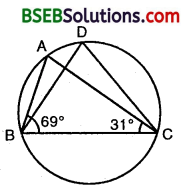Solution:
In ∆ ABC,
∠BAC + ∠ABC + ∠BCA = 180°
∠BAC + 69° + 31° = 180°
∠BAC = 180° – (69° + 31°) = 180° – 100° = 80°
Since angles in the same segment are equal.
∴ ∠BDC = ∠BAC = 80°.

Question 5.
In figure, A, B, C and D are four points on a circle. AC and BD intersect at a point such that ∠BEC – 130° and , ∠ECD = 20°. Find ∠BAC.Solution:Question 6.
ABCD is a cyclic quadrilateral whose diagonals intersect at a point E. If ∠DBC = 70°, ∠BAC is 30% find ∠BCD. Further, if AB = BC, find ∠ECD.
Solution:
∠BDC = ∠BAC
[Angles in the same segment]
⇒ ∠BDC = 30° [∵ ∠BAC = 30° (given)]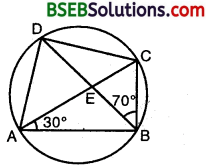In A BCD, we have ∠BDC + ∠DBC + ∠BCD = 180°
[Sum of ∠s of a A]
⇒ 30° + 70° + ∠BCD = 180° [∵ ∠DBC = 70°, ∠BDC = 30°]
⇒ ∠BCD = 180° – 30° – 70° = 80°
If AB = BC, then ∠BCA = ∠BAC = 30° [Angles opp. to equal sides in a A are equal]
Now, ∠ECD = ∠BCD – ∠BCE
= 80° – 30° = 50°
[∵ ∠BCD = 80° (found above) and ∠BCE = ∠BCA = 30°]
Hence, ∠BCD = 80° and ∠ECD = 50°.

Question 7.
If diagonals of a cyclic quadrilateral are diameters of the circle through the vertices of the . quadrilateral, prove that it is a rectangle.
Solution:
Diagonals AC and BD of a cyclic quadrilateral are diameters of the circle through the vertices A, B, C and D of the quad. ABCD.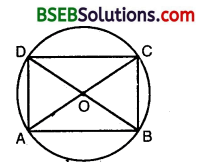To prove : Quadrilateral ABCD is a rectangle.
Solution:
Since all the radii of the same circle are equal. OA = OB = OC = OD
∴ OA = OC = 12 AC
⇒ OB = OD= 12 BD .
AC = BD
∴ The diagonals, of the quadrilateral ABCD are equal and bisect each other.
⇒ Quadrilateral ABCD is a rectangle.

Question 8.
If the non-parallel sides of a trapezium are equal, prove that it is cyclic.
Solution:
Given: Non-parallel sides AD and BC of a trapezium are equal.
To prove : ABCD is a cyclic trapezium.
Construction : Draw DE ± AB and CF ⊥ AB.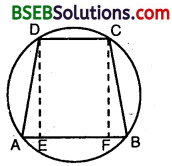Proof: In order to prove that ABCD is a cyclic trapezium, it is sufficient to prove that ∠B + ∠D = 180°.
In As DEA and CFB, we have
∠DEA = ∠CFB [Each = 90°]
DE = CF [Distance between two || lines is always equal]
∴ By RHS criterion of congruence, we have
∆ DEA ≅ ∆ CFB
⇒ 90° + ∠ADE = 90° + ∠BCF
⇒ ∠EDC + ∠ADE = ∠FCD + ∠BCF [∵ ∠EDC = 90° and ∠FCD = 90°]
⇒ ∠D = ∠C
Thus, ∠A = ∠B and ∠C = ∠D
∴ ∠A + ∠B + ∠C + ∠D = 360° [∵ Sum of the angles of a quad, is 360°]
⇒ 2∠B + 2∠D = 360°
⇒ ∠B + ∠D = 180°
Hence, ABCD is a cyclic trapezium.

Question 9.
Two circles intersect at p two points B and C. Through B, two line segments ABD and PBQ are drawn to intersect the circles at A, D and P, Q respectively (see figure).Prove that ∠ACP = ∠QCD.
Solution: Since angles in the same segment are equal.
∴ ∠ACP = ∠ABP … (1)
and ∠QCD = ∠QBD … (2)
Also ∠ABP = ∠QBD …(3) [∵ Vertically opp. angles]
From (1), (2) and (3),
we have ∠ACP = ∠QCD.

Question 10.
If circles are drawn taking two sides of a triangle as diameters, prove that the point of intersection of these circles lie on the third side.
Solution:
Given : Two circles are drawn with sides AB and AC of A ABC as diameters. The circle intersect at D.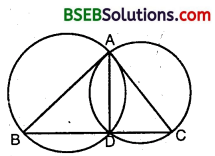To prove : D lies on BC.
Construction : Join A and D.
Proof : Since AB and AC are the diameters of the two circles [Given]
∴ ∠ADB = 90° [Angles in a semi-circle]
and, ∠ADC = 90° [Angles in a semi-circle]
⇒ BDC is a straight line.
Hence, D lies on BC.

Question 11.
ABC and ADC are two right triangles with common hypotenuse AC. Prove that ∠CAD = ∠CBD.
Solution:
∆s ABC and ADC are right ∠d with common hypotenuse AC. Draw a circle with AC as diameter passing through B and D. Join BD.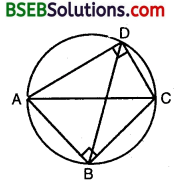[∵ Angles in the same segment are equal]

Question 12.
Prove that a cyclic parallelogram is a rectangle.
Solution:
Given : ABCD is a parallelogram inscribed in circle.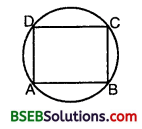To prove : ABCD is a rectangle. Proof : Since ABCD is a cyclic quadrilateral. ‘
∴ ∠A + ∠C = 180°
But ∠A = ∠C
From (1) and (2), we have
∠A = ∠C = 90°
Similarly, ∠B + ∠D = 90°
∴ Each angle of ABCD is of 90°
Hence, ABCD is a rectangle.

## Bihar Board Class 9th Maths Chapter 10 Circles Ex 10.5 Textbooks for Exam Preparations

Bihar Board Class 9th Maths Chapter 10 Circles Ex 10.5 Textbook Solutions can be of great help in your Bihar Board Class 9th Maths Chapter 10 Circles Ex 10.5 exam preparation. The BSEB STD 9th Maths Chapter 10 Circles Ex 10.5 Textbooks study material, used with the English medium textbooks, can help you complete the entire Class 9th Maths Chapter 10 Circles Ex 10.5 Books State Board syllabus with maximum efficiency.

## FAQs Regarding Bihar Board Class 9th Maths Chapter 10 Circles Ex 10.5 Textbook Solutions

#### Can we get a Bihar Board Book PDF for all Classes?

Yes you can get Bihar Board Text Book PDF for all classes using the links provided in the above article.

## Important Terms

Bihar Board Class 9th Maths Chapter 10 Circles Ex 10.5, BSEB Class 9th Maths Chapter 10 Circles Ex 10.5 Textbooks, Bihar Board Class 9th Maths Chapter 10 Circles Ex 10.5, Bihar Board Class 9th Maths Chapter 10 Circles Ex 10.5 Textbook solutions, BSEB Class 9th Maths Chapter 10 Circles Ex 10.5 Textbooks Solutions, Bihar Board STD 9th Maths Chapter 10 Circles Ex 10.5, BSEB STD 9th Maths Chapter 10 Circles Ex 10.5 Textbooks, Bihar Board STD 9th Maths Chapter 10 Circles Ex 10.5, Bihar Board STD 9th Maths Chapter 10 Circles Ex 10.5 Textbook solutions, BSEB STD 9th Maths Chapter 10 Circles Ex 10.5 Textbooks Solutions,
Share: